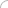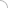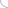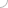# Random Access Memory interview questions and answers

## Random Access Memory interview questions and answers

### 1. How does a RAM function? 2. What are differences between RAM and ROM? 3. What is a flash memory? 4. What are the different RAM standards/versions? 5. What is the purpose of RAM in a computer? 6. What kind of memory is a RAM categorized as? 7. How does the CPU and the RAM communicate? 8. What is the function of the control lines in RAM? 9. What are the different types package forms? 10. How is the total memory on a ram stick calculated? 11. What is bursting? 12. What is buffered RAM? 13. What is pipelining in reference to RAM? 14. What is SO-DIMM in reference to RAM? 15. Why are Wait states used? 16. What are banks in reference to RAM? 17. What are the functions of the RAM IC`s? 18. What is the difference between RAM and cache memory? 19. How is the maximum addressable memory calculated? 20. What does the 400 Mhz signify in a ram specification? 21. What are the various capacities in which a RAM is available? 22. How can a DDR1 ram be identified from a DDR2 ram module physically? 23. Explain the basic concepts and hierarchy of memory? 24. Why is RAM also known as volatile memory? 25. State the differences between DDR1, 2 and 3. 26. What is SDRAM? 27. What is RDRAM? 28. What is feRAM? 29. What is ECC in reference to ram? 30. How does ECC works? 31. What is tCL timing? 32. What is tRCD timing? 33. What is tRP timing? 34. What is tRAS timing? 35. In a Ram description DDR 2 1066mhz “2.5-3-3-8” what do the numbers in quotes signify? 36. Explain the command rate in reference to RAM? 37. What is the tRC timing? 38. What is the tRTW/tRWT timing? 39. Explain the tRDA? 40. What is the limit of RAM capacity in a 32bit OS? 41. What is shadow ram? 42. Explain what is the memory wall problem? 43. Explain the dual channel architecture? 44. Explain the triple channel architecture? 45. What is RAM parity? 46. What is the Unified memory architecture? Explain. 47. What is virtual memory? 48. What is a phase change memory? 49. Explain the refresh operation in a dynamic RAM? 50. Can two different RAM speed modules be used together? (adsbygoogle = window.adsbygoogle || []).push({}); Write your comment - Share Knowledge and Experience (adsbygoogle = window.adsbygoogle || []).push({}); (adsbygoogle = window.adsbygoogle || []).push({});Interview questionsLatest MCQs » General awareness - Banking » ASP.NET » PL/SQL » Mechanical Engineering » IAS Prelims GS » Java » Programming Language » Electrical Engineering » English » C++ » Software Engineering » Electronic Engineering » Quantitative Aptitude » Oracle » English » Finance Home | About us | Sitemap | Contact us | We are hiring © Copyright 2016. All Rights Reserved. Terms of use  |  Follow us on Facebook!